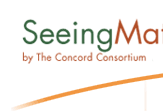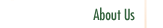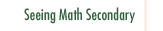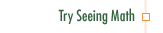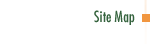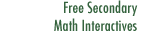Site Map About Us About The Concord Consortium Evaluation Methods Behind Seeing Math Philosophy Behind Seeing Math™ Research Behind Seeing Math People Behind Seeing Math™ Teachscape PBS TeacherLine Seeing Math Elementary Video Case Studies Moderated Discussion Interactive Software & Related Activities Effective Questioning in the Mathematics Classroom Formative Assessment in the Mathematics Classroom Number & Operations: Division with Remainders Number & Operations: The Magnitude of Fractions Number & Operations: Broken Calculator Geometry: 2D and 3D Figures Geometry: Calculating the Area of a Triangle Data Analysis and Probability: Using Data to Make Predictions Data Analysis and Probability: Measures of Center Pre-Algebra: Pan Balance Equations Pre-Algebra: Patterns and Functions Seeing Math Secondary Video Case Studies Moderated Discussion Interactive Software & Related Activities Proportional Reasoning Linear Functions Transformations of Linear Functions Linear Equations Systems of Linear Equations Quadratic Functions Transformations of Quadratic Functions Quadratic Equations Data Analysis Secondary Course Structure Resources Contact Us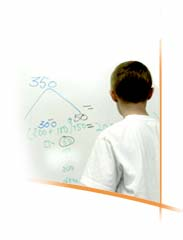©2005 The Concord Consortium
Home | About Us | Seeing Math™ Elementary | Seeing Math™ Secondary | Try Seeing Math™ | Resources | Contact Us | Site Map